### Parameter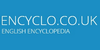A numerical descriptive measure used in statistics, economics and modelling where it refers to a variable. Coefficients that control model process rates but do not change in the run of a model.

### parameter(Learning Modules / Mathematics / Modelling projectiles) Input variable or constant.

### Parameter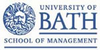A characteristic of a population (e.g. the mean age of all nurses studying the MSc in Nursing by distance learning with the RCN Institute)
Found on http://www.bath.ac.uk/catalogues/information/glossary/

### Parameteras the most fundamental object being considered, then defining functions with fewer variables from the main one by means of currying. Sometimes it is useful to consider all functions with certain parameters as parametric family, i.e. as an indexed family of functions. Examples from probability theory are given further below. ===Parametric equation...
Found on http://en.wikipedia.org/wiki/Parameter

### Parameter• (n.) The ratio of the three crystallographic axes which determines the position of any plane; also, the fundamental axial ratio for a given species. • (n.) Specifically (Conic Sections), in the ellipse and hyperbola, a third proportional to any diameter and its conjugate, or in the parabola, to any abscissa and the corresponding ordinat...
Found on http://thinkexist.com/dictionary/meaning/parameter/

### Parameter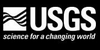(1) A statistical parameter is a numerical characteristic about the population of interest (Freedman et al. 1970:301); (2) A model parameter is a numerical quantity that mediates the relationships between variables in a model (Starfield and Bleloch 1980:4).
Found on http://www.encyclo.co.uk/local/21070

### Parameter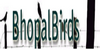(1) A statistical parameter is a numerical characteristic about the population of interest (Freedman et al. 1978301); (2) A model parameter is a numerical quantity that mediates the relationships between variables in a model (Starfield and Bleloch 19864).
Found on http://www.encyclo.co.uk/local/22216

### parameter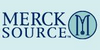(pә-ram´ә-tәr) in a mathematical expression, a constant that distinguishes specific cases, having a definite fixed value in one case but different values in other cases. in statistics, a value that specifies one of the members of a family of probability distributions, such as the mean or ...
Found on http://www.encyclo.co.uk/local/21001

### parameter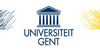(para- + meter) a variable whose measure is indicative of a quantity or function that cannot itself be precisely determined by direct methods; e.g., blood pressure and pulse rate are parameters of cardiovascular function, and the level of glucose in blood and urine is a parameter of carbohydrate metabolism.
Found on http://users.ugent.be/~rvdstich/eugloss/DIC/dictio64.html

### Parameter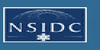1) A term used loosely by many meteorologists for almost any meteorological quantity or element. 2) An arbitrary constant or variable so appearing in a mathematical expression that changing it gives various cases of the phenomenon represented.
Found on http://nsidc.org/arcticmet/glossary/parameter.html

### parameterparametric quantity noun a constant in the equation of a curve that can be varied to yield a family of similar curves
Found on https://www.encyclo.co.uk/local/20974

### Parameter[computer programming] In computer programming, a parameter is a special kind of variable, used in a subroutine to refer to one of the pieces of data provided as input to the subroutine.{efn|1=In this article, the term `subroutine` refers to any subroutine-like construct, which have different names and slightly different meanings depending ...
Found on http://en.wikipedia.org/wiki/Parameter_(computer_programming)

### ParameterPa·ram'e·ter noun [ Prefix para- + -meter : confer French paramètre .] 1. (a) (Math.) A term applied to some characteristic magnitude whose value, invariable as long as one and the same function, curve, surface, etc., is considered, serves to distinguish t...
Found on http://www.encyclo.co.uk/webster/P/20

### Parameter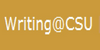A coefficient or value for the population that corresponds to a particular statistic from a sample and is often inferred from the sample.
Found on http://writing.colostate.edu/guides/guide.cfm?guideid=50

### Parameter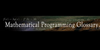A constant in a mathematical program, not subject to choice in the decision problem, but one that could vary outside the control of the decisions. Examples are supplies, demands, loss factors, exponents and coefficients in polynomial functions (of the decision variables). Not all coefficients are parameters, as many are zero by the logic of the mo....
Found on http://glossary.computing.society.informs.org/index.php?page=P.html

### parameter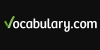a constant in the equation of a curve that can be varied
Found on https://www.vocabulary.com/lists/1842734

### parameter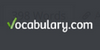a constant in the equation of a curve that can be varied
Found on https://www.vocabulary.com/lists/311755

### ParameterA measurable or derived variable representedby the data (e.g. air temperature, snow depth, relative humidity.
Found on http://www.encyclo.co.uk/local/20130

### Parameter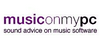A MIDI value seen in the envelope of a particular instrument that alters the integrity of the sound itself. Common parameters include pitch bend, sustain, volume, and reverb
Found on http://www.encyclo.co.uk/local/20532

### Parameter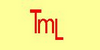A MIDI value seen in the envelope of a particular instrument that alters the integrity of the sound itself. Common parameters include pitch bend, sustain, volume, and reverb. A variable value that affects some aspect of a device's performance. Characteristic elements of a sound that are usually programmable in a synthesizer or other MIDI device.

### Parameter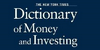A model is a combination of variables, such as GDP growth, and coefficients which multiply these variables. The coefficients are often estimated from the data. The coefficients are called parameters.
Found on http://www.duke.edu/~charvey/Classes/wpg/bfglosp.htm

### Parameter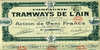A model is a combination of variables, such as GDP growth, and coefficients which multiply these var
Found on http://www.encyclo.co.uk/local/22402

### Parameter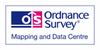A quantity that is fixed for the case in question, but may vary in other cases.
Found on http://www.encyclo.co.uk/local/20195

### ParameterA representation that characterizes a part of a model (e.g. a growth rate), the value of which is determined outside of the model. See: exogenous variable.
Found on http://www.encyclo.co.uk/local/20047

### Parameter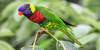A statistical parameter is a numerical characteristic about the population of interest (Freedman et al. 1978:301); (2) A model parameter is a numerical quantity that mediates the relationships between variables in a model (Starfield and Bleloch 1986:4).
Found on http://www.encyclo.co.uk/local/22030
No exact match found